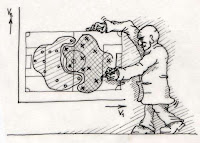## Pages

### Forming cluster with categorical dataMost of people who know clustering might be aware about the back-end algorithm of the clustering(K-Means), provided the data is continuous.

Do you know how the clusters are being formed if the data is categorical?

Read the article to explore !

We will explain both the techniques here :

1) Clustering with Continuous Data

2) Clustering with Attributes ( Categorical Data)

### 1. Clustering with Continuous Data

We are taking very simple example with only six observation to explain the concept.

Sample data with employees Age and Income.

In clustering method we club observations with similarities( proximity). We use distance method to club the observation.

How do we measure distance?

Assume we have two data points on 2D-Plane  A(28,20) and B(31,10). Lets estimate the distance.

The above method of calculating distance is call Euclidean Distance. We need to calculate the distance between each pair of observations.

### Clustering Method (Basic)

We begin by considering each observation as a separate cluster. Merge the pair of the observations with minimum distance as one cluster and leaving n-1 cluster for the next step. We repeat this process until a single cluster is formed.

Since Suresh and Amit has the minimum distance, they will form a first cluster.

In order to delve deeper in clustering analysis, please follow our below enlisted blogs:
_________________________________________________________________________

_________________________________________________________________________

In this particular blog, we are focusing upon the clustering with categorical variables.

### 2. Clustering with Attributes ( Categorical Data)

Let's assume a situation where you will have the data in category format as given below :

 Name Age Category Income Category Marital Status Rajat 25-30 20-25 M Vinod 30-35 10-15 M Amit 25-30 15-20 S Suresh 20-25 10-15 S Dinesh 30-35 10-15 M Ganesh 35-40 10-15 M

Here we cannot calculate the distance using Euclidean Distance formula mathematically, so we find out another method to do so.

We check the number of attributes which are same for particular pair of observations and this is considered as "Alternative Distance Measure".

Distance measure for attributes

For example : Rajat and Vinod are same only in one attributes (Marital Status). So,

D( Rajat, Vinod) = 1- (1/3) = 2/3

The Distance Matrix

It consists the extent of common attributes between each pair of observations.

Please find below SAS code to calculate the  Distance Matrix on Categorical Data

/***********************************************************************/;;
* Macro to create "Distance Matrix" on Attributes ;
/***********************************************************************/;;
options symbolgen mprint mlogic ;
%let dataset=base_data   ; /* Basic dataset */

%let id=Name ; /* Primary key variable for observations  */
%let distance_matrix=output ; /* Need to name the distance matrix file */

%macro distance;

proc transpose data=&dataset.(obs=0)  out=varlist ;
var _all_ ;
run;

proc sql ;

select distinct _name_ into : varlist separated by " " from varlist
where _name_ not in ( "&id." );
quit ;

proc transpose data=&dataset.  out=t_dataset  ;

id &id. ;
var &varlist. ;
run;

data t_dataset;

set t_dataset;
varcnt+1;
run;

proc sql ;     /* Storing number of observation and number of variables into macro variables */

select count(*) into : nobs from &dataset. ;
select count(*) into : nvar from t_dataset;
quit;

data _null_;

set &dataset. ;
%do i=1 %to &nobs. ;
if _n_ = &i. then call symput("var&i.",strip(&id.));
%end;
run;

data &distance_matrix. ;

length id \$15. ;
%do i=1 %to &nobs. ;
id="&&var&i." ;output;
%end;
run;

%put &var1 &var2 ;

%do i=1 %to &nobs. ;
%do j=1 %to &nobs. ;

proc sql ;

create table a.for_distance as select a.&&var&i. as a, b.&&var&j. as b
from t_dataset as a,
t_dataset as b
where a.varcnt=b.varcnt ;
quit;

data a.for_distance;

set a.for_distance;
if a=b then value=1 ; else value=0;
run;

proc sql noprint;

select 1-(sum(value)/&nvar.) into : distance_val&j. from a.for_distance ;
quit;

%end;

data &&var&i. ;

%do j=1 %to &nobs. ;
&&var&i.=&&distance_val&j. ;output;
%end;
run;

%end;

data &distance_matrix.;

set &distance_matrix. ;
%do t=1 %to &nobs. ;
set &&var&t. ;
%end ;
run;

proc delete data=

%do t=1 %to &nobs. ;
&&var&t.
%end ;
;
run;

proc print data=&distance_matrix. ;

run;

%mend ;

%distance ;
*************************************** End *****************************;;;

Distance Matrix using above code on our sample database.

Code for clustering using Distance Matrix

proc cluster data=&distance_matrix. method=centroid
pseudo outtree=tree;
id id;
var Rajat--Ganesh;
run;

goptions reset=all;

ods listing close;
ods pdf file="mygraph3.pdf";

goptions vsize=8in htext=1pct htitle=2.5pct;

axis1 order=(0 to 1 by 0.2);
proc tree data=tree n=4 out=out;
height _rsq_;
id id;
run;

ods pdf close;

ods listing;

Tree Output :

Enjoy reading our other articles and stay tuned with us.

Kindly do provide your feedback in the 'Comments' Section and share as much as possible.